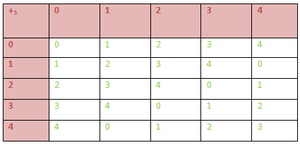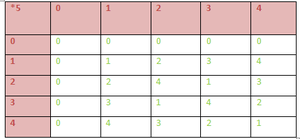# Mathematics | Rings, Integral domains and Fields

• Last Updated : 19 May, 2022

Prerequisite – Mathematics | Algebraic Structure

Ring – Let addition (+) and Multiplication (.) be two binary operations defined on a non empty set R. Then R is said to form a ring w.r.t addition (+) and multiplication (.) if the following conditions are satisfied:

1. (R, +) is an abelian group ( i.e commutative group)
2. (R, .) is a semigroup
3. For any three elements a, b, cR the left distributive law a.(b+c) =a.b + a.c and the right distributive property (b + c).a =b.a + c.a holds.

Therefore a non- empty set R is a ring w.r.t to binary operations + and . if the following conditions are satisfied.

1. For all a, bR, a+bR,
2. For all a, b, cR a+(b+c)=(a+b)+c,
3. There exists an element in R, denoted by 0 such that a+0=a for all a4. For every aR there exists an yR such that a+y=0. y is usually denoted by -a
5. a+b=b+a for all a, bR.
6. a.bR for all a.bR.
7. a.(b.c)=(a.b).c for all a, b8. For any three elements a, b, cR a.(b+c) =a.b + a.c and (b + c).a =b.a + c.a. And the ring is denoted by (R, +, .).

Some Examples –

1. (, + ) is a commutative group .(, .) is a semi-group. The distributive law also holds. So, ((, +, .) is a ring.

2. Ring of Integers modulo n: For a n[Tex]\mathbb{N}   [/Tex]letbe the classes of residues of integers modulo n. i.e={).
(, +) is a commutative group ere + is addition(mod n).
(, .) is a semi group here . denotes multiplication (mod n).
Also the distributive laws hold. So ((, +, .) is a ring.
3. The set S = {0, 1, 2, 3, 4} is a ring with respect to operation addition modulo 5 & multiplication modulo 5.(S,+5) is an Abelian Group. From the above 1st composition table we can conclude that (S,+5) satisfies –

• Closure :  a ∈ S ,b ∈ S  => a +5 b ∈ S ;  ∀ a,b ∈ S
• Associativity : (a+5b)+5c = a+5(b+5c) ; ∀ a,b,c ∈ S.
• Existence of identity 0 : (a+5b)+5c = a+5(b+5c) ; ∀ a,b,c ∈ S.
• Existence of inverse: Inverse of 0, 1, 2, 3, 4 are 0, 4, 3, 2 , 1 respectively &
• Commutative :  (a+5b) = (b+5a) ;  ∀ a,b ∈ S

2. (S,*5) is an Semi Group. From the above 2nd composition table we can conclude that (S,*5) satisfies :

• Closure : a ∈ S ,b ∈ S  => a *5 b ∈ S ;  ∀ a,b ∈ S
• Associativity :  (a*5b)*5c = a*5(b*5c) ; ∀ a,b,c ∈ S

3. Multiplication is distributive over addition :

(a) Left Distributive :   ∀ a, b, c ∈ S :

a*5 (b +5 c)

= [ a * (b + c) ] mod 5

= [a*b + a*c] mod 5

= (a *5 b) +5 (a *5 c)

⇒ Multiplication modulo 5 is distributive over addition modulo 5.

Similarly , Right Distributive law can also be proved.

So, we can conclude that (S,+,*) is a Ring.

Many other examples also can be given on rings like (, +, .), (, +, .) and so on.

Before discussing further on rings, we define Divisor of Zero in A ringand the concept of unit

Divisor of Zero in A ring –
In a ring R a non-zero element is said to be divisor of zero if there exists a non-zero element b in R such that a.b=0 or a non-zero element c in R such that c.a=0 In the first case a is said to be a left divisor of zero and in the later case a is said to be a right divisor of zero . Obviously if R is a commutative ring then if a is a left divisor of zero then a is a right divisor of zero also .

Example – In the ring (, +, .)are divisors of zero sinceand so on .
On the other hand the rings (, +, .), (, +, .), (, +, .) contains no divisor of zero .

Units –
In a non trivial ring R( Ring that contains at least to elements) with unity an element a in R is said to be an unit if there exists an element b in R such that a.b=b.a=I, I being the unity in R. b is said to be multiplicative inverse of a.

Some Important results related to Ring:

1. If R is a non-trivial ring(ring containing at least two elements ) withunity I then I0.
2. If I be a multiplicative identity in a ring R then I is unique .
3. If a be a unit in a ring R then its multiplicative inverse is unique .
4. In a non trivial ring R the zero element has no multiplicative inverse .

Types of Ring :

1. Null Ring : The singleton set : {0} with 2 binary operations ‘+’ & ‘*” defined by :
0+0 = 0 & 0*0 = 0 is called zero/ null ring.
2. Ring with Unity : If there exists an element in R denoted by 1 such that :
1*a = a* 1 = a ; ∀ a ∈ R, then the ring is called Ring with Unity.
3. Commutative Ring : If the multiplication in the ring R is also commutative, then ring is called a commutative ring.
4. Ring of Integers : The set I of integers with 2 binary operations ‘+’ & ‘*’ is known as ring of Integers.
5.  Boolean Ring : A ring whose every element is idempotent, i.e. , a2 = a ; ∀ a ∈ R
Now we introduce a new concept Integral Domain.

Integral Domain – A non -trivial ring(ring containing at least two elements) with unity is said to be an integral domain if it is commutative and contains no divisor of zero ..

Examples –
The rings (, +, .), (, +, .), (, +, .) are integral domains.
The ring (2, +, .) is a commutative ring but it neither contains unity nor divisors of zero. So it is not an integral domain.

Next we will go to Field .

Field – A non-trivial ring R with unity is a field if it is commutative and each non-zero element of R is a unit . Therefore a non-empty set F forms a field .r.t two binary operations + and . if

1. For all a, bF, a+bF,
2. For all a, b, cF a+(b+c)=(a+b)+c,
3. There exists an element in F, denoted by 0 such that a+0=a for all a4. For every aR there exists an yR such that a+y=0. y is usually denoted by (-a)
5. a+b=b+a for all a, bF.
6. a.bF for all a.bF.
7. a.(b.c)=(a.b).c for all a, b8. There exists an element I in F, called the identity element such that a.I=a for all a in F
9. For each non-zero element a in F there exists an element, denoted byin F such that=I.
10. a.b =b.a for all a, b in F .
11. a.(b+c) =a.b + a.c for all a, b, c in F

Examples – The rings (, +, .), (, + . .) are familiar examples of fields.

Some important results:

1. A field is an integral domain.
2. A finite integral domain is a field.
3. A non trivial finite commutative ring containing no divisor of zero is an integral domain

My Personal Notes arrow_drop_up
Recommended Articles
Page :